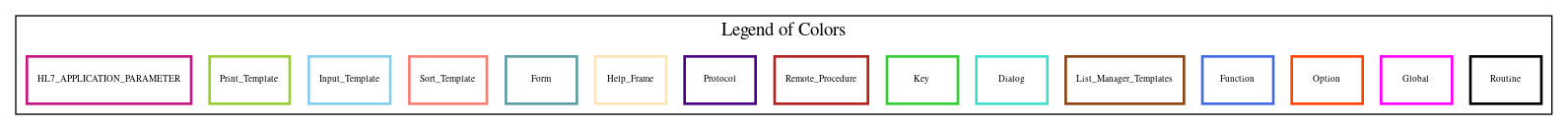Home   Package List   Routine Alphabetical List   Global Alphabetical List   FileMan Files List   FileMan Sub-Files List   Package Component Lists   Package-Namespace Mapping
Routine: ENFABAL1

# Routine: ENFABAL1

## Information

ENFABAL1 ;WIRMFO/SAB-MAINTAIN FILE 6915.9 FAP BALANCES (cont);1.12.98

## Source Information

Source file <ENFABAL1.m>

## Call Graph### Call Graph Total: 3

Package Total Call Graph
Engineering 2 (\$\$AFA,\$\$FUND)^ENFAR5A  \$\$EOM^ENUTL
VA FileMan 1 \$\$GET1^DIQ

## Caller Graph

### Legends:#### Package Component Superscript legend

 action A extended action Ea event driver Ed subscriber Su protocol O limited protocol LP run routine RR broker B edit E server Se print P screenman SM inquire I### Caller Graph Total: 1

Package Total Caller Graph
Engineering 1 ENFABAL

## Entry Points

SUM ; sum FAP transactions for period
; called from RECALC^ENFABAL
; input
; ENDTR - month to recalculate (FileMan date)
; output
; ^TMP(\$J,"R",station,fund,sgl)=net \$ activity
FVST ; compare file 6915.9 vs. transactions
; input
; ENDTR - month to recalculate (FileMan date)
; ^TMP(\$J,"R",station,fund,sgl)=net \$ activity from recalc
; output -
; problems where net activity is not equal in
; ^TMP(\$J,"P",station,fund,sgl)=net from file^net from recalc

## External References

Name Field # of Occurrence
\$\$GET1^DIQ FVST+10, FVST+13, FVST+17
\$\$AFA^ENFAR5A SUM+20
\$\$FUND^ENFAR5A SUM+24
\$\$EOM^ENUTL SUM+9

## FileMan Files Accessed Via FileMan Db Call

FileNo Call Tags
^ENG(6915.9 - [#6915.9] GET1^DIQ
6915.91 GET1^DIQ
6915.911 GET1^DIQ

## Global Variables Directly Accessed

Name Line Occurrences  (* Changed,  ! Killed)
^ENG( SUM+17, SUM+19, SUM+27, SUM+28, SUM+29, SUM+30, SUM+31, SUM+36
^ENG(6914.3 - [#6914.3] SUM+11, SUM+12
^ENG(6915.2 - [#6915.2] SUM+21
^ENG(6915.9 - [#6915.9] FVST+9, FVST+12, FVST+16, FVST+18, FVST+19, FVST+20, FVST+21, FVST+22
^TMP(\$J SUM+34*, SUM+38*, SUM+39*, FVST+24, FVST+25*

## Local Variables

### Legend:

 >> Not killed explicitly * Changed ! Killed ~ Newed

Name Field # of Occurrence
ENAMT SUM+6~, SUM+27*, SUM+28*, SUM+29*, SUM+30*, SUM+31*, SUM+32, SUM+34, SUM+38, SUM+39
ENDA SUM+6~, SUM+11*, SUM+12
ENDA("F?" SUM+18*, SUM+19*, SUM+20, SUM+24, SUM+27, SUM+28, SUM+29, SUM+30, SUM+31, SUM+36
ENDA("FA" SUM+20*, SUM+21, SUM+24
ENDT SUM+6~, SUM+16*, SUM+17*, SUM+19
ENDTE SUM+6~, SUM+9*, SUM+17
>> ENDTR SUM+8, FVST+18, FVST+19
ENDTS SUM+6~, SUM+8*, SUM+9, SUM+16
ENFAPTY SUM+6~
ENFAPTY( SUM+13*, SUM+25
ENFAY3 SUM+6~, SUM+21*, SUM+23, SUM+25
ENFILE SUM+6~, SUM+15*, SUM+17, SUM+19, SUM+20, SUM+23, SUM+24, SUM+27, SUM+28, SUM+29
SUM+30, SUM+31, SUM+33, SUM+35, SUM+36
ENFUND SUM+7~, SUM+23*, SUM+24*, SUM+26, SUM+34, SUM+37, SUM+39, FVST+7~, FVST+13*, FVST+24
FVST+25
ENFUNDNW SUM+7~, SUM+36*, SUM+37, SUM+38
ENI SUM+7~, FVST+7~
ENI(1 FVST+9*, FVST+10, FVST+12, FVST+13, FVST+16, FVST+17, FVST+18, FVST+19, FVST+20, FVST+21
FVST+22
ENI(2 FVST+12*, FVST+13, FVST+16, FVST+17, FVST+18, FVST+19, FVST+20, FVST+21, FVST+22
ENI(3 FVST+15*, FVST+16*, FVST+17, FVST+18, FVST+19, FVST+20, FVST+21, FVST+22
ENPM FVST+7~, FVST+19*, FVST+20
ENPMI FVST+7~, FVST+20*, FVST+22
ENSGL SUM+7~, SUM+25*, SUM+26, SUM+34, SUM+38, SUM+39, FVST+7~, FVST+17*, FVST+24, FVST+25
ENSMI FVST+7~, FVST+18*, FVST+21
ENSN SUM+7~, SUM+21*, SUM+22*, SUM+34, SUM+38, SUM+39, FVST+7~, FVST+10*, FVST+24, FVST+25
ENX SUM+7~, SUM+29*
ENY0 SUM+7~, SUM+12*, SUM+13
PAMT FVST+7~, FVST+22*, FVST+23, FVST+25
RAMT FVST+7~, FVST+24*, FVST+25
SAMT FVST+7~, FVST+21*, FVST+23*, FVST+25
U SUM+13, SUM+21, SUM+23, SUM+25, SUM+27, SUM+28, SUM+29, SUM+30, SUM+31, SUM+36
FVST+21, FVST+22, FVST+24, FVST+25